Function Repository Resource:

# SphericalVoronoiDiagram

Show the spherical Voronoi diagram of a set of points on a sphere

Contributed by: Jan Mangaldan
 ResourceFunction["SphericalVoronoiDiagram"][{p1,…,pn}] generates a three-dimensional plot representing the spherical Voronoi diagram of the points pi.

## Details and Options

The points pi are assumed to lie in a unit sphere centered at the origin.
ResourceFunction["SphericalVoronoiDiagram"] takes the same options as Graphics3D, with the following additions and changes:
 Boxed False whether to draw the bounding box EdgeStyle Automatic graphics directives to specify the style for the edges "PointStyle" Automatic graphics directives to specify the style for the generating points "ShowOriginalPoints" False whether to show the generating points "SphereStyle" Automatic graphics directives to specify the style for the sphere

## Examples

### Basic Examples (1)

Spherical Voronoi diagram of the result of SpherePoints:

 In:=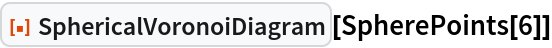Out=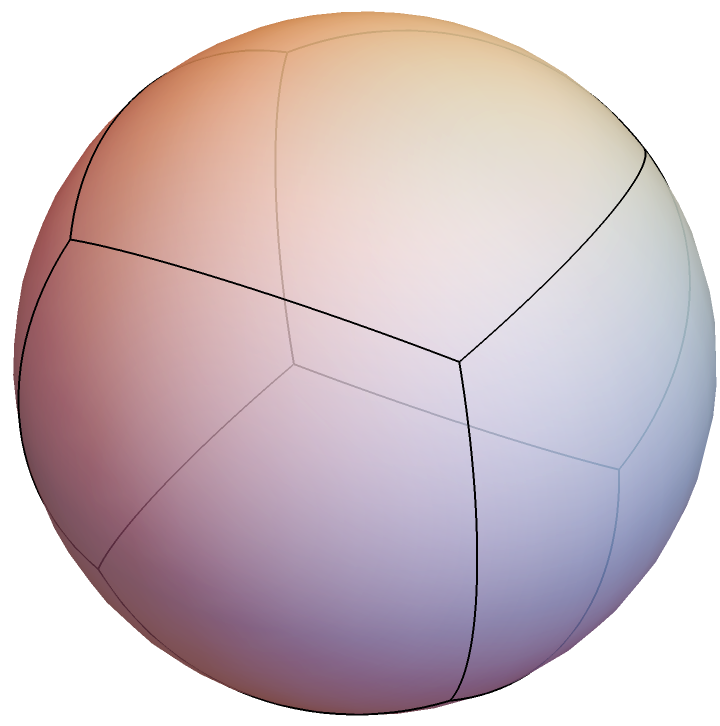### Scope (2)

Spherical Voronoi diagram of two and three points:

 In:=Out=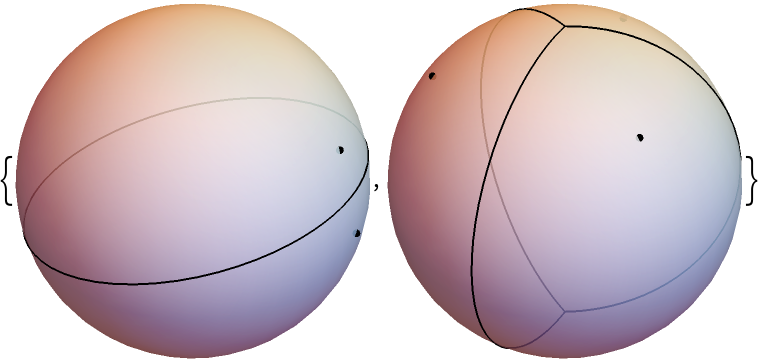Spherical Voronoi diagram of randomly generated points:

 In:=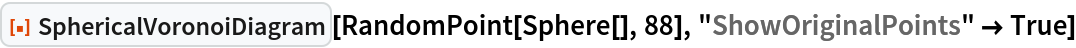Out=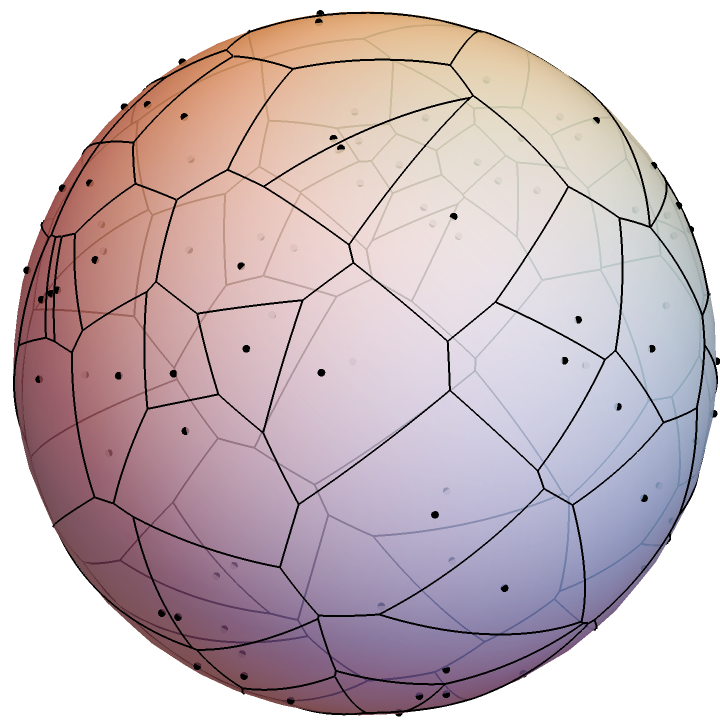### Options (4)

#### EdgeStyle (1)

Change the style of the edges:

 In:=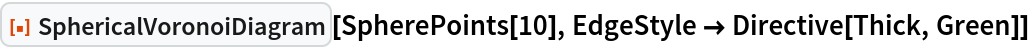Out=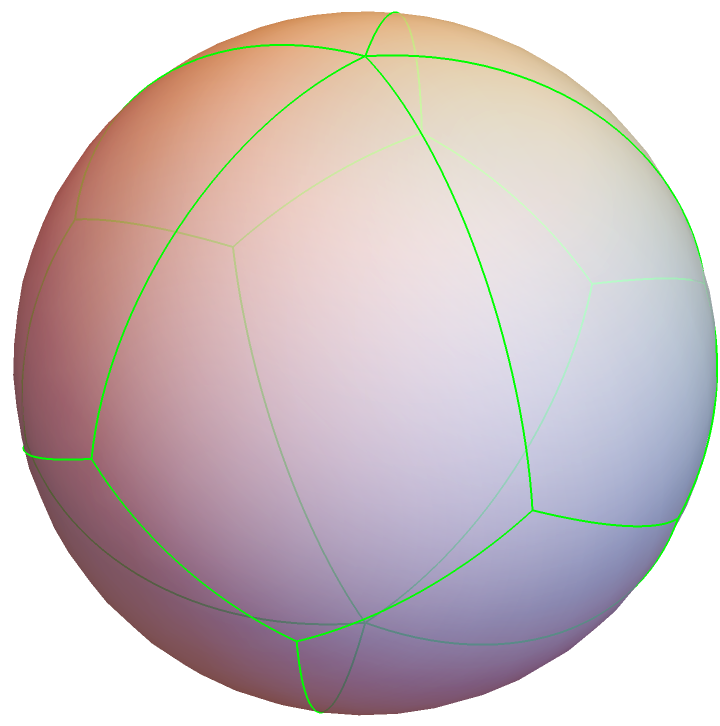#### PointStyle (1)

Change the style of the generating points:

 In:=Out=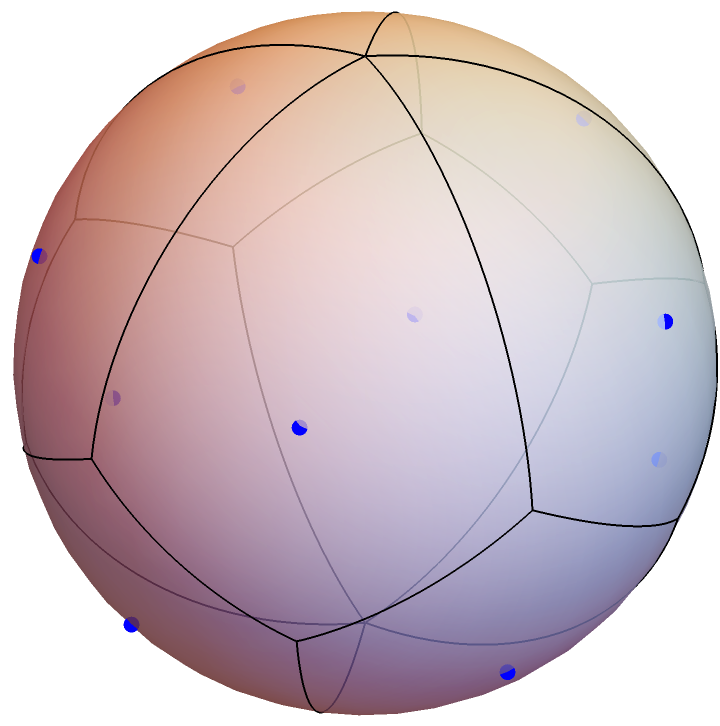#### ShowOriginalPoints (1)

Show the spherical Voronoi diagram with and without the generating points:

 In:=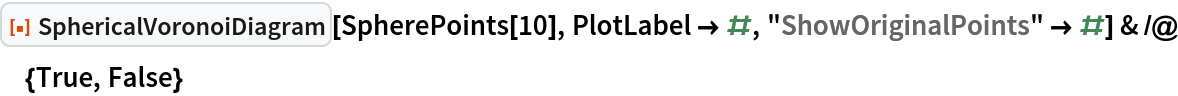Out=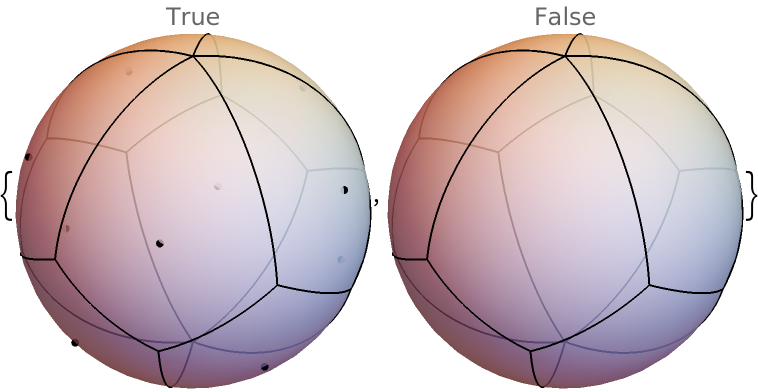#### SphereStyle (1)

Change the style of the sphere:

 In:=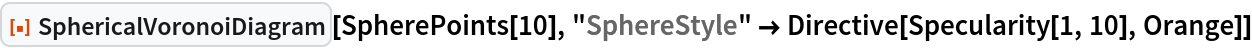Out=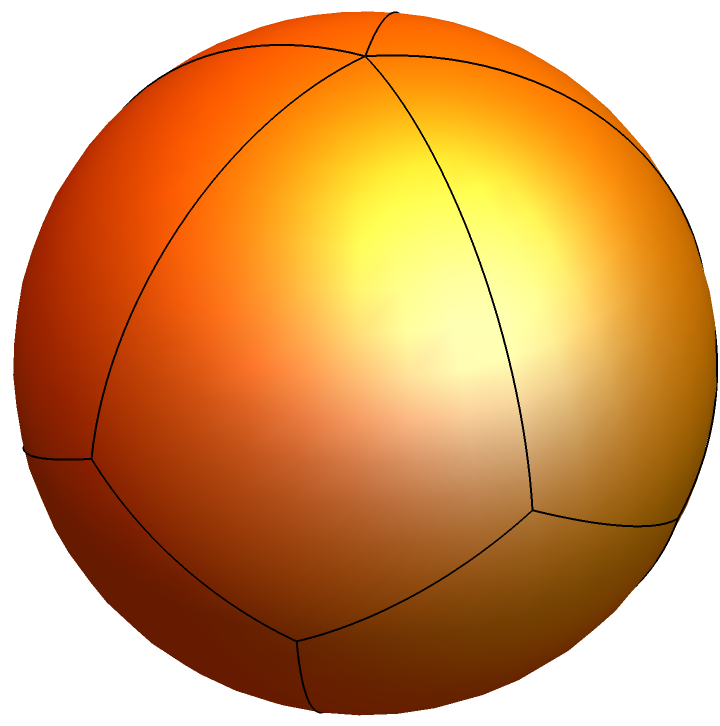### Applications (2)

Use tubes and spheres to render the spherical Voronoi diagram and its generating points:

 In:=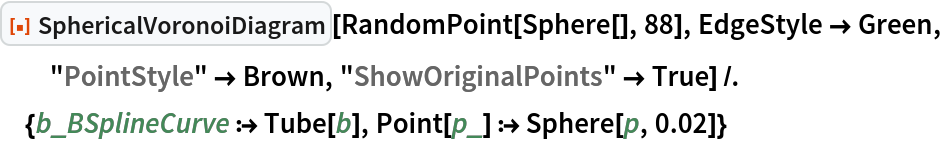Out=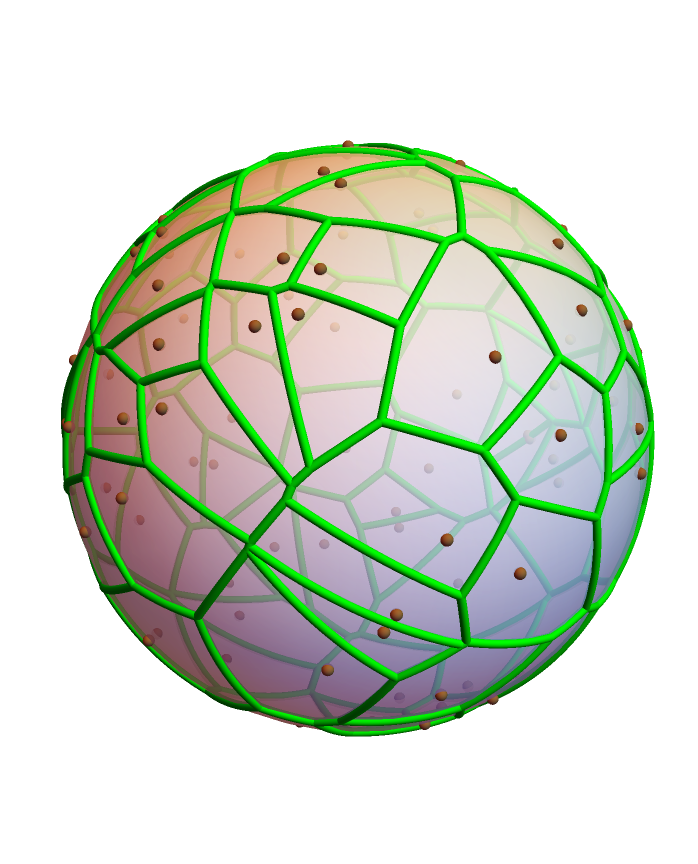Locations of the state capitals of the contiguous United States:

 In:=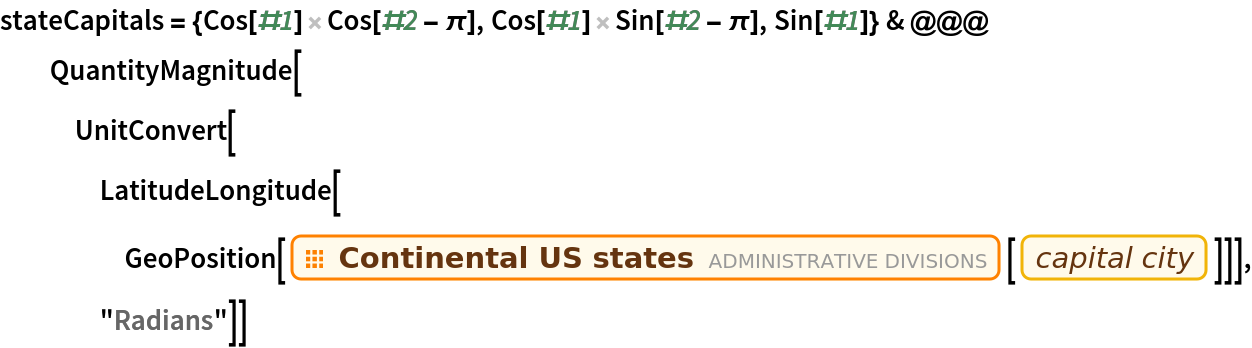Out=Display them in a spherical Voronoi diagram:

 In:=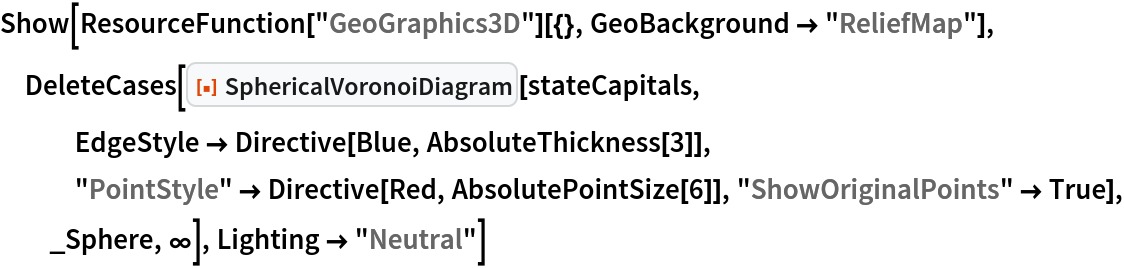Out=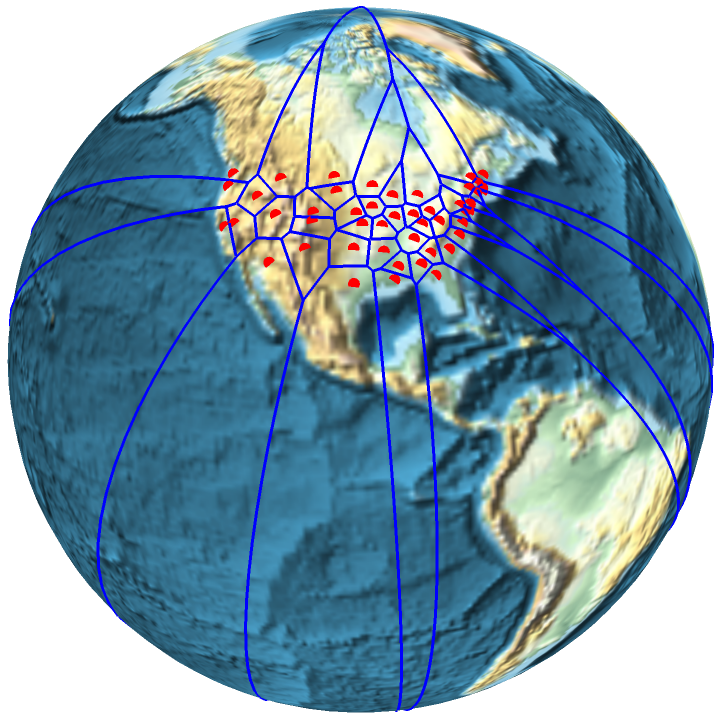### Neat Examples (1)

Spherical Voronoi diagram of the vertices of a pentakis dodecahedron:

 In:=Out=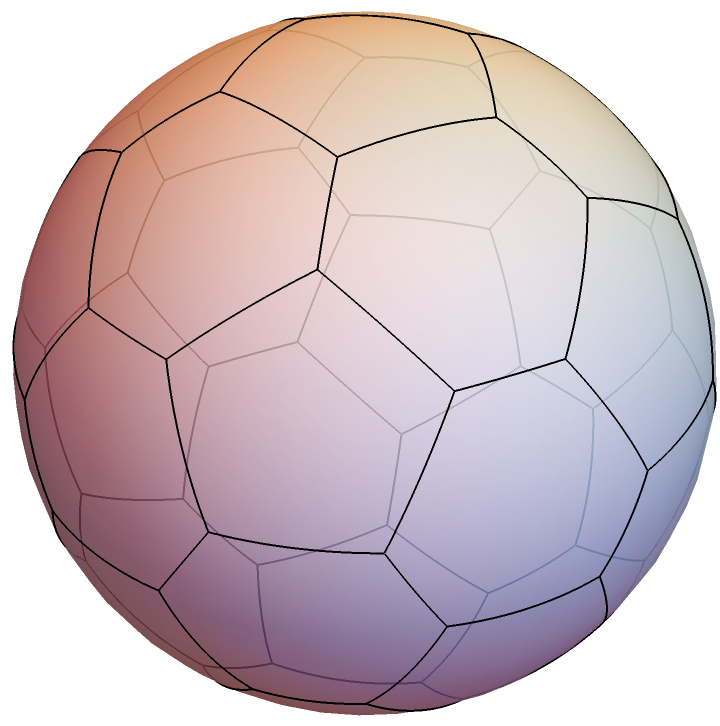## Version History

• 1.0.0 – 14 June 2021